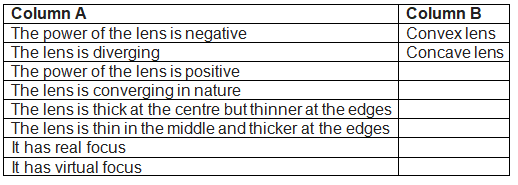# Light Class 10 Problems and solutions

In this page we have Light Problems and Solutions | Class 10 physics Questions . Hope you like them and do not forget to like , social shar and comment at the end of the page.
a. Multiple Choice Questions
c. Match the column

## Multiple Choice Quetsions

Question 1
Magnification produced by a rear view mirror fitted in vehicles
(a) is less than one
(b) is more than one
(c) is equal to one
(d) can be more than or less than one depending upon the position of the object in front of it.
Question 2
Which of the following statements is true?(1 Marks)
(a) The image formed in a plane mirror is real
(b) The image in the plane mirror is of the same size as the object
(c) An object is placed at a distance of 20 cm in front of plane mirror; the image formed would be 40 cm from the object
(d) The image formed in a plane mirror is laterally inverted.
Question 3
A full length image of a distant tall building can definitely be seen by using
(a) a concave mirror
(b) a convex mirror
(c) a plane mirror
(d) both concave as well as plane mirror
Question 4
What kind of mirror is used in vehicles to see the traffic on the rear side?
(a) Convex mirror
(b) Plane mirror
(c) Concave mirror
Question 5
Which of the following is true for concave mirror?
(a) When the object is at infinity, the image is formed at focal point.
(b) It forms both highly enlarged and highly diminished images
(c) When the object is placed at center of curvature, image is real, inverted and positioned at C only.
(d) Image formed is always virtual and erect.
Question 6
Rays from sun converge at a point 15 cm in front of concave mirror. Where the object should be placed so that size of its image is equal to the size of the object?
(a) 15 cm in front of the mirror
(b) 30 cm in front of the mirror
(c) Between 15 cm and 30 cm in front of the mirror
(d) more than 30 cm in front of the mirror

Question 7
In torches, search lights and headlights of vehicles bulb is placed
(a) Between pole and focus of the reflector
(b) Very near to the focus of reflector
(c) Between the focus and center of curvature of the reflector
(d) At the center of curvature of the reflector
Question 8
In which of the following, the image of an object placed at infinity will be highly diminished and point sized?
(a) Concave mirror only
(b) Convex mirror only
(c) Convex lens only
(d) Concave mirror, convex mirror, concave lens and convex lens
Question 9(1 Marks)
John is standing in front of a special mirror. He finds image of his head smaller, the middle portion of his body the same size and the legs bigger. The following is the order of combinations for the special mirror from the top
(a) Plane, convex, concave
(b) Convex, concave, plane
(c) Concave, plane, convex
(d) Convex, plane and concave
Question 10(1 Marks)
Which of the following statements is true?
(a) A convex lens has 5 dioptre power having a focal length 0.20 m
(b) A convex lens has -5 dioptre power having a focal length 0.20 m
(c) A concave lens has 5 dioptre power having a focal length 0.20 m
(d) A concave lens has -5 dioptre power having a focal length 0.20 m

Question 1
(a) What is lateral inversion? Explain by giving suitable example.
(b) How would the letter ‘F’ appear in front of plane mirror?
Question 2
At what distance from a concave mirror of focal length 10 cm should an object 2 cm long be placed in order to get erect image 6 cm tall.

### Match the column## Long answer type questions Reflection of light Problems

Question 1
State the relation between the object distance, image distance and focal length of the mirror.
Question 2
Draw ray diagrams showing the image formation by a convex mirror when an object is placed at
(a) infinity
(b) at finite distance from the mirror
Question 3
Draw ray diagrams showing the image formation by a concave mirror when an object is placed
(a) at focal point
(b) at center of curvature
(c) Between focal point and center of curvature
Question 4
Write down the five uses of each convex and concave mirror?
Question 5
A convex lens forms a real and inverted image of a needle at a distance of 50 cm from it. Where is the needle placed in front of the convex lens if the image is equal to the size of the object? Also, find the power of the lens.
Question 6
A Calculate the focal length of convex lens which produces a virtual image at a distance of 25 cm of an object placed 10cm in front of it.
Question 7
What is lateral shift and optical density?
Question 8
What is scientific name for short sightness and far sightness ? What types of lens are used to correct them?

Question 9
Define the following terms used in the reflection of light
(a) Incident ray
(b) Normal
(c) Reflected ray
(d) Angle of incidence
(e) Angle of reflection

## Summary

This Light Problems and Solutions | Class 10 physics Questions with answers is prepared keeping in mind the latest syllabus of CBSE . This has been designed in a way to improve the academic performance of the students. If you find mistakes , please do provide the feedback on the mail.